# I.E. Irodov Solutions on Kinematics

Students can find the solutions of the Problems In General Physics I.E. Irodov - Kinematics on this page. The branch of physics that deals with motion with respect to space and time without considering the cause of that motion, is known as kinematics. This is an important topic for the JEE Main exam. The important topics in kinematics include particle, motion, displacement and distance, average speed and average velocity, etc. It also includes velocity and acceleration of a point, average vectors of velocity, and acceleration of a point, distance covered by a point, angular velocity and angular acceleration of a solid body, acceleration of a point expressed in projections on the tangent, and the normal to a trajectory, etc.

By referring to these solutions, students can achieve higher marks in the JEE exam. Students are recommended to go through these solutions thoroughly before the exams to score well and improve their problem-solving abilities.

### I.E. Irodov Solutions on Kinematics

1. A motorboat going downstream overcame a raft at a point A; T = 60 min later it turned back and after some time passed the raft at a distance l = 6.0 km from the point A. Find the flow velocity assuming the duty of the engine to be constant.

Solution:

1. Let v0 be the stream velocity and v’ the velocity of motorboat w.r.t. water. The motorboat reached point B while going downstream with velocity (v0 + v’) and then returned with velocity (v’ - v0) and passed the raft at point C. Let t be the time for the raft (which flows with stream velocity v0) to move from point A to C, during which the motorboat moves from A to B and then from B to C.

Therefore l/v0 = T + ((v0 + v’)T - l)/(v’ - v0)

On solving we get v0 = l/2T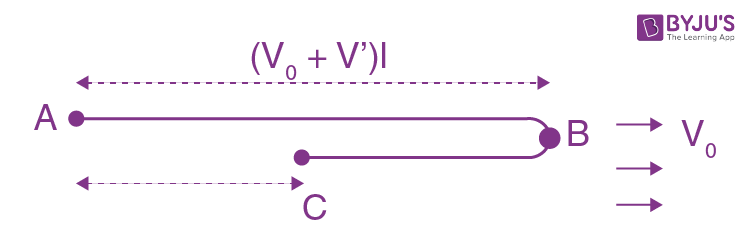2. A point traversed half the distance with a velocity v0. The remaining part of the distance was covered with velocity v1 for half the time, and with velocity v2 for the other half of the time. Find the mean velocity of the point averaged over the whole time of motion.

Solution:

1. Let s be the total distance traversed by the point and t1 the time taken to cover half the distance. Further, let 2t be the time to cover the rest half of the distance.

Therefore s/2 = v0t1

or t1 = s/2v0 …(1)

And s/2 = (v1 + v2)t

Or 2t = s/(v1 + v2) …(2)

Hence the sought average velocity

<v> = s/t1 + 2t

= s/([s/2v0] + [s/(v1 + v2)])

= 2v0(v1 + v2)/(v1 + v2 + 2v0)

3. A car starts moving rectilinearly, first with acceleration w = 5.0 m/s2 (the initial velocity is equal to zero), then uniformly, and finally, decelerating at the same rate w, comes to a stop. The total time of motion equals t = 25 s. The average velocity during that time is equal to (v) = 72 km per hour. How long does the car move uniformly?

Solution:

1. As the car starts from rest and finally comes to a stop, and the rate of acceleration and deceleration are equal, the distances, as well as the times taken, are the same in these phases of motion. Let ∆t be the time for which the car moves uniformly. Then the acceleration/ deceleration time is (t - ∆t)/2 each. So,

v t = 2{½ w (t - ∆t)2/4} + w(t - ∆t) ∆t/2

∆t2 = t2 - (4vt/w)

Hence ∆t = t√(1 - 4v/wt) = 15 s

4. A point moves rectilinearly in one direction. Fig. 1.1 shows the distance s traversed by the point as a function of the time t.

Using the plot find:

1. (a) the average velocity of the point during the time of motion;

2. (b) the maximum velocity;

3. (c) the time moment to at which the instantaneous velocity is equal to the mean velocity averaged over the first to seconds.

4.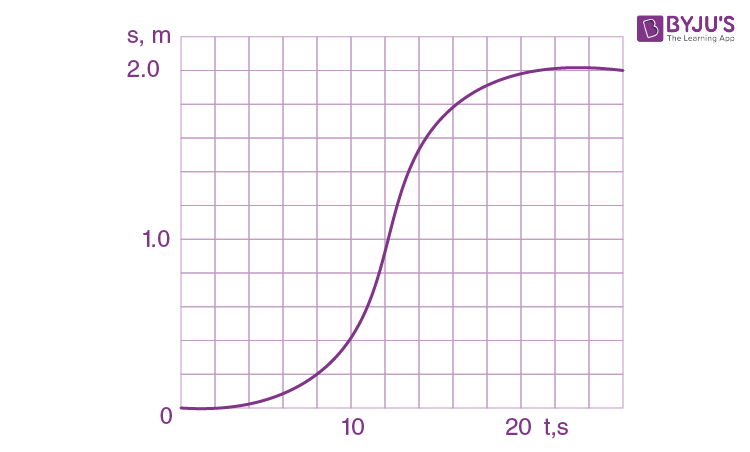Solution:

1.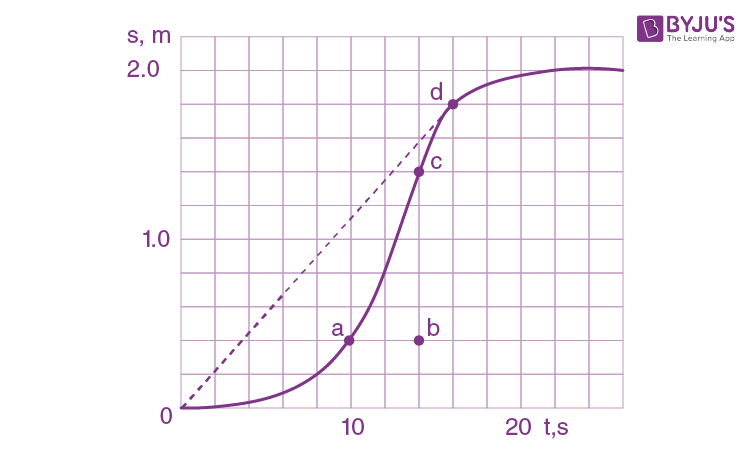(a) Sought average velocity

v = s/t = 200cm/20s

= 10cm/s

(b) For the maximum velocity, ds/dt should be maximum. From the figure ds/dt is maximum for all points on the line ac, thus the sought maximum velocity becomes average velocity for the line ac and is equal to :

bc/ab = 100cm/4s = 25 cm/s

(c) Time t0 should be such that corresponding to it the slope ds/dt should pass through the point O (origin), to satisfy the relationship ds/dt = s/t0. From figure the tangent at point d passes through the origin and thus corresponding time t = t0 = 16 s

5. Two particles, 1 and 2, move with constant velocities v1 and v2. At the initial moment, their radius vectors are equal to r1 and r2. How must these four vectors be interrelated for the particles to collide?

Solution:

1. Let the particles collide at the point A (Fig.), whose position vector is $\vec{r}$

(say).If t be the time taken by each particle to reach at point A, from triangle law of vector addition :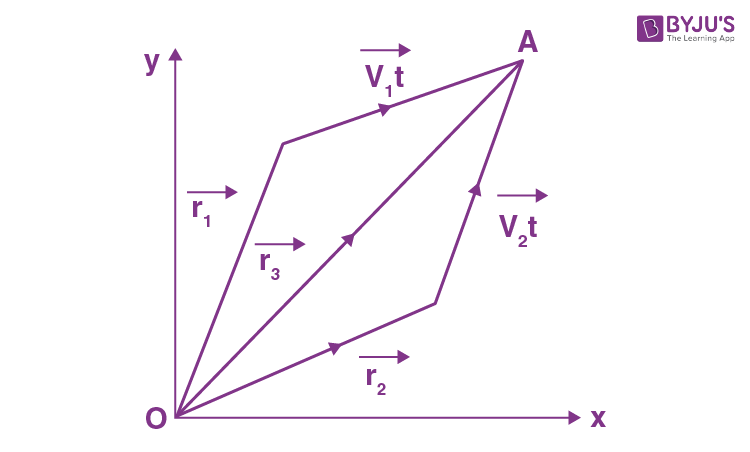$\vec{r_{3}}= \vec{r_{1}}+\vec{v_{1}}t$

So $\vec{r_{1}}-\vec{r_{2}}= (\vec{v_{2}}-\vec{v_{1}})t$ ..(1)

Therefore$t = \frac{\left | \vec{r_{1}}-\vec{r_{2}} \right |}{\left | \vec{v_{2}}-\vec{v_{1}} \right |}$ …(2)

From equations (1) and (2)

$\vec{r_{1}}=\vec{r_{2}}-(\vec{v_{2}}-\vec{v_{1}})\frac{\left |\vec{r_{1}}-\vec{r_{2}} \right |}{\left | \vec{v_{2}}-\vec{v_{1}} \right |}$

Or $\frac{\vec{r_{1}}-\vec{r_{2}}}{\left |\vec{r_{1}}-\vec{r_{2}} \right |} = \frac{\vec{v_{2}}-\vec{v_{1}}}{\left |\vec{v_{2}}-\vec{v_{1}} \right |}$

which is the sought relationship.

6. A ship moves along the equator to the east with velocity vo = 30 km/hour. The southeastern wind blows at an angle φ = 600 to the equator with velocity v = 15 km/hour. Find the wind velocity v' relative to the ship and the angle φ' between the equator and the wind direction in the reference frame fixed to the ship.

Solution:

1.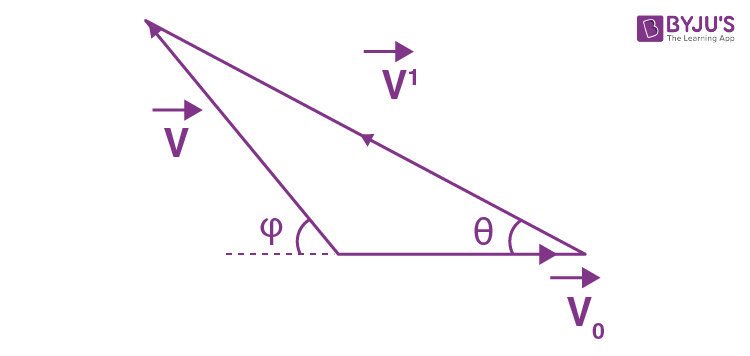We have $\vec{v'} = \vec{v}-\vec{v_{0}}$

From the vector diagram of eq (1) and using the properties of triangle

v’ = √(v02 + v2 + 2v0v cos φ) = 39.7 km/hr (2)

And v’/sin (π - φ) = v/sin θ

Or sin θ = v sin φ/v’

θ = sin-1(v sin φ/v’)

Using (2) and putting the values of v and d

θ = 19.10

7. Two swimmers leave point A on one bank of the river to reach point B lying right across on the other bank. One of them crosses the river along the straight line AB while the other swims at right angles to the stream and then walks the distance that he has been carried away by the stream to get to point B. What was the velocity u of his walking if both swimmers reached the destination simultaneously? The stream velocity v0 = 2.0 km/hour and the velocity v’ of each swimmer with respect to water equals 2.5 km per hour.

Solution:

1. Let one of the swimmer (say 1) cross the river along AB, which is obviously the shortest path. Time taken to cross the river by the swimmer 1.

t1 = d/√(v’2- v02), (where AB = d is the width of the river.) ..(1)

For the other swimmer (say 2), which follows the quickest path, the time taken to cross the river.

t2 = d/v’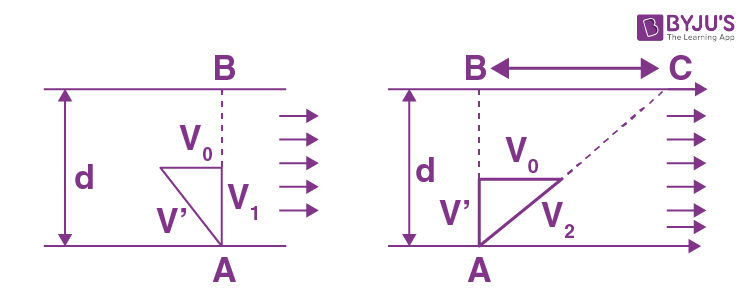In the time t2 drifting of the swimmer 2 becomes

x = v0t2 = v0d/v’ (using eq. 2) ..(3)

If t3 be the time for swimmer 2 to walk the distance x to come from C to B, then

t3 = x/u = v0d/v’u (using eq. 3) ..(4)

according to the problem t1 = t2 + t3

Or d/√(v’2 - v02) = d/v’ + v0d/v’u

On solving we get u = v0/((1 - v02)/v’2)-1/2 - 1

= 3 km/hr

8. Two boats, A and B, move away from a buoy anchored at the middle of a river along the mutually perpendicular straight lines: the boat A along the river, and the boat B across the river. Having moved off an equal distance from the buoy the boats returned. Find the ratio of times of motion of boats TA/TB if the velocity of each boat with respect to water is η = 1.2 times greater than the stream velocity.

Solution:

1. Let l be the distance covered by boat A along the river as well as by the boat B across the river. Let v0 be the stream velocity and v' the velocity of each boat with respect to water. Therefore time taken by the boat A in its journey

tA = l/(v’ + v0) + l/(v’ - v0)

tB = l/√(v’2 - v02) + l/√(v’2 - v02)

= 2l/√(v’2 - v02)

Hence tA/tB = v’/√(v’2 - v02)

= η/√(η2 - 1) (where η = v’/v)

On substitution tA/tB = 1.8

9. A boat moves relative to water with a velocity which is n = 2.0 times less than the river flow velocity. At what angle to the stream direction must the boat move to minimize drifting?

Solution:

1. Let v0 be the stream velocity and v’ the velocity of boat with respect to water.

v0/v’ = η = 2>0, some drifting of boat is inevitable.

Let $\vec{v'}$ make an angle θ with flow direction. Then the time taken to cross the river

t = d/v’ sin θ (where d is the width of the river)

In this time interval, the drifting of the boat

x = (v’ cos θ + v0)t

= (v’ cos θ + v0) d/v’ sin θ

= (cot θ + η cosec θ)d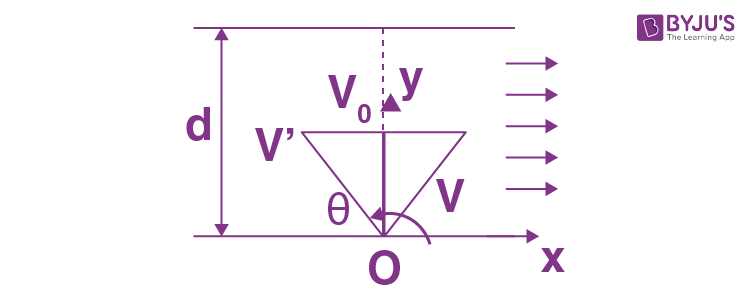For xmin(minimum drifting)

d/dθ (cot θ + η cosec θ) = 0, which yields

cos θ = -1/η

= -1/2

θ = 1200

10. Two bodies were thrown simultaneously from the same point: one, straight up, and the other, at an angle of θ = 60° to the horizontal. The initial velocity of each body is equal to vo = 25 m/s. Neglecting the air drag, find the distance between the bodies t = 1.70 s later.

Solution:

1. The solution of this problem becomes simple in the frame attached with one of the bodies. Let the body thrown straight up be 1 and the other body be 2, then for the body 1 in the frame of 2 from the kinematic equation for constant acceleration:

$\vec{r_{12}}=\vec{r_{0(12)}}+\vec{v_{0(12)}}t+\frac{1}{2}\vec{w_{12}}t^{2}$

So, $\vec{r}_{12}=\vec{v}_{0(12)}t$

$\left | \vec{r}_{12}\right |=\left | \vec{v}_{0(12)} \right |t$

But $\left | \vec{v}_{01} \right |=\left | \vec{v}_{02} \right |=v_{0}$

So from the properties of triangle

v0(12) = √(v02 + v02 - 2v0v0 cos (π/2 - θ0)

Hence the sought distance

$\left | \vec{r_{12}}\right |$ = v0√(2(1 - sin θ)) t

= 22 m

11. Two particles move in a uniform gravitational field with an acceleration g. At the initial moment the particles were located at one point and moved with velocities v1 = 3.0 m/s and v2 = 4.0 m/s horizontally in opposite directions. Find the distance between the particles at the moment when their velocity vectors become mutually perpendicular.

Solution:

1.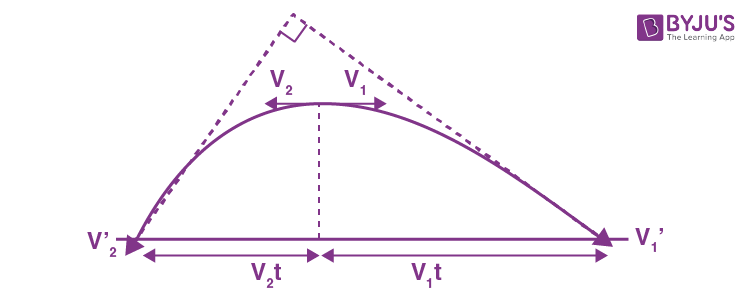Let the velocities of the particles (say $\vec{v_{1}}'$ and $\vec{v_{2}}'$ ) becomes mutually perpendicular after time t. Then their velocities becomes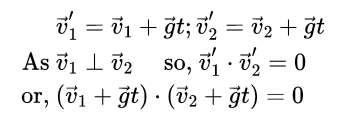Or -v1v2 + g2t2 = 0

Hence t = √(v1v2)/g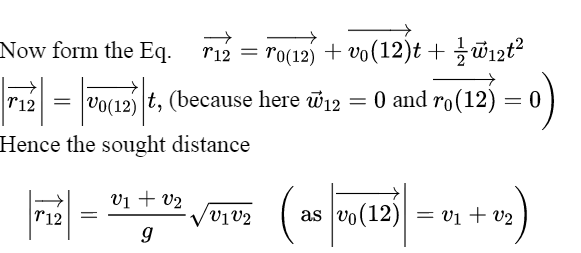12. Three points are located at the vertices of an equilateral triangle whose side equals a. They all start moving simultaneously with velocity v constant in modulus, with the first point heading continually for the second, the second for the third, and the third for the first. How soon will the points converge?

Solution:

1. From the symmetry of the problem all the three points are always located at the vertices of equilateral triangles of varying side length and finally meet at the centroid of the initial equilateral triangle whose side length is a, in the sought time interval (say t).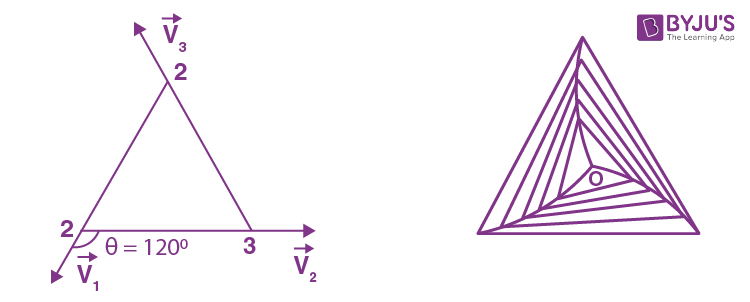Let us consider an arbitrary equilateral triangle of edge length l (say). Then the rate by which 1 approaches 2, 2 approaches 3, and 3 approaches 1, becomes

-dl/dt = v - v cos (2π/3)

On integrating

$-\int_{0}^{a}dl = \frac{3v}{2}\int_{0}^{t}dt$

a = (3/2) vt

So t = 2a/3v

13. Point A moves uniformly with velocity v so that the vector v is continually "aimed" at point B which in its turn moves rectilinearly and uniformly with velocity u< v. At the initial moment of time v u and the points are separated by a distance l. How soon will the points converge?

Solution:

1. Let us locate the points A and B at an arbitrary instant of time (Fig.).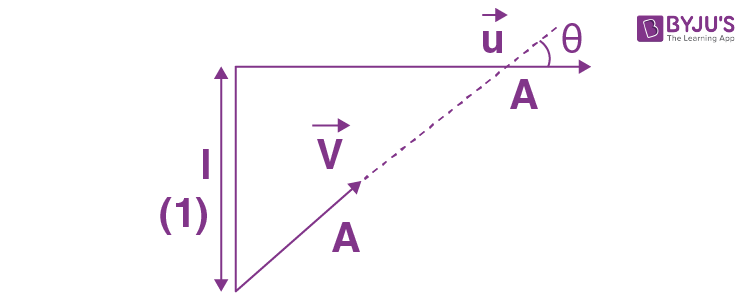If A and B are separated by the distance s at this moment, then the points converge or point A approaches B with velocity -ds/dt = v - u cos α where angle α varies with time.

On integrating

$-\int_{l}^{0}ds = \int_{0}^{t}(u - v \cos \alpha )dt$

Where T is the sought time.

$l= \int_{0}^{t}(u - v \cos \alpha )dt$ ..(1)

As both A and B cover the same distance in x direction during the sought time interval, so the other condition which is required, can be obtained by the equation

$\Delta x= \int v_{x}dt$

So uT = $\int_{0}^{t}v\cos \alpha dt$ ..(2)

Solving (1) and (2), we get T = ul/(v2 - u2)

One can see that if u = v, or u < v, point A cannot catch B.

14. A train of length 1 = 350 m starts moving rectilinearly with constant acceleration w = 3.0.10-2 m/s2; t = 30 s after the start the locomotive headlight is switched on (event 1) , and T = 60 s after that event the tail signal light is switched on (event 2) . Find the distance between these events in the reference frames fixed to the train and to the Earth. How and at what constant velocity V relative to the Earth must a certain reference frame K move for the two events to occur in it at the same point?

Solution:

1. In the reference frame fixed to the train, the distance between the two events is obviously equal to l. Suppose the train starts moving at time t = 0 in the positive x direction and take the origin (x = 0) at the head coordinate of first event in the earth’s frame is light o f the train at t = 0. Then the coordinate of first event in the earth’s frame is

x1 = ½ wt2

And similarly the coordinate of the second event is

x2 = ½ w(t+T)2 - l

The distance between the two events is obviously

x1 - x2 = l - wT(t + T/2)

= 0.242 km

In the reference frame fixed frame on the earth.

For the two events to occur at the same point in the reference frame K, moving with constant velocity V relative to the earth, the distance travelled by the frame in the time interval T must be equal to the above distance.

Thus VT = l - wT(t + T/2)

So V = 1/T - w(t + T/2)

= 4.03 m/s

The frame K must clearly be moving in a direction opposite to the train so that if (for example) the origin of the frame coincides with the point x1 on the earth at time t, itcoincides with the point x2 at time t + T.

15. An elevator car whose floor-to-ceiling distance is equal to 2.7 m starts ascending with constant acceleration 1.2 m/s2; 2.0 s after the start a bolt begins falling from the ceiling of the car. Find:

1. (a) the bolt's free fall time;

Solution:

1. (a) One good way to solve the problem is to work in the elevator’s frame having the observer at its bottom (Fig.).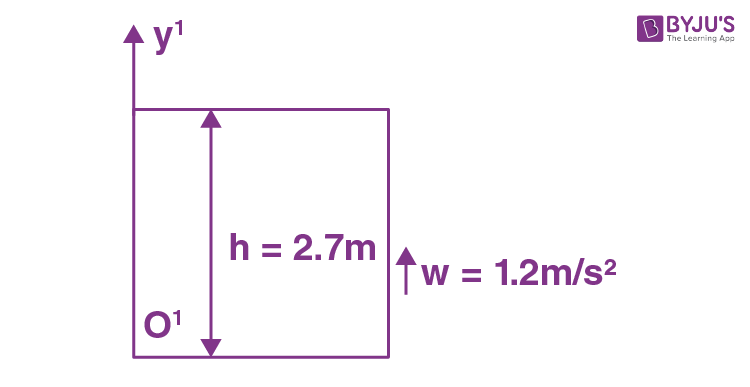Let us denote the separation between floor and ceiling by h = 2.7 m and the acceleration of the elevator by w = 1.2m/s2

From the kinematical formula

y = y0 + v0yt + ½ wyt2 ..(1)

Here y = 0, y0 = +h, v0y = 0

And wy = bolt(y) - wele(y)

= -g - w

= -(g + w)

So, 0 = h + ½ (-(g + w))t2

Or t = √(2h/(g + w))

= 0.7 s

(b) At the moment the bolt loses contact with the elevator, it has already acquired the velocity equal to elevator, given by

v0 = (1.2)2

= 2.4 m/s

In the reference frame attached with the elevator shaft (ground) and pointing the y axis upward, we have for the displacement of the bolt,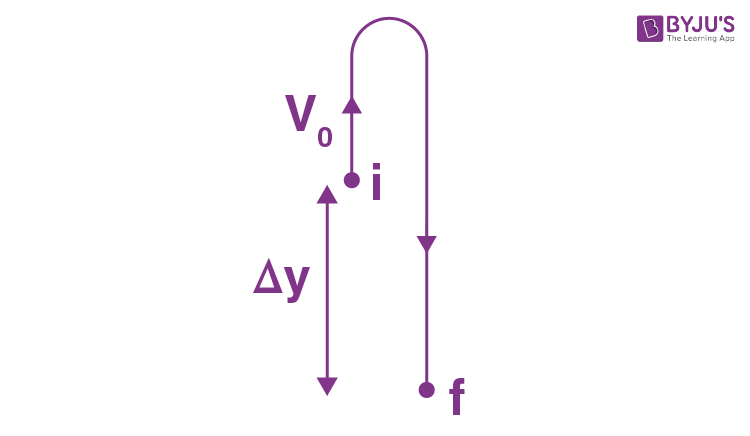∆y = v0yt + ½ wyt2

= v0t + ½ (-g)t2

Or ∆y = (2.4)(0.7) + ½ (-9.8)(0.7)2

= -0.7 m

Hence the bolt comes down or displaces downward relative to the point, when it loses contact with the elevator by the amount 0.7 m (Fig.). Obviously the total distance covered by the bolt during its free fall time.

s = |∆y| + 2(v02/2g)

= 0.7 m + 2.42/9.8 m

= 1.3 m

16. Two particles, 1 and 2, move with constant velocities v1 and v2 along two mutually perpendicular straight lines toward the intersection point O. At the moment t = 0 the particles were located at the distances l1 and l2 from the point O. How soon will the distance between the particles become the smallest? What is it equal to?

Solution:

1. Let the particle 1 and 2 be at points B and A at t = 0 at the distances l1 and l2 from intersection point O . Let us fix the inertial frame with the particle 2. Now the particle 1 moves in relative to this reference frame with a relative velocity $\vec{v}_{12}= \vec{v}_{1}-\vec{v}_{2}$ and its trajectory is the straight line BP. Obviously, the minimum distance between the particles is equal to the length of the perpendicular AP dropped from point A on to the straight line BP (Fig.).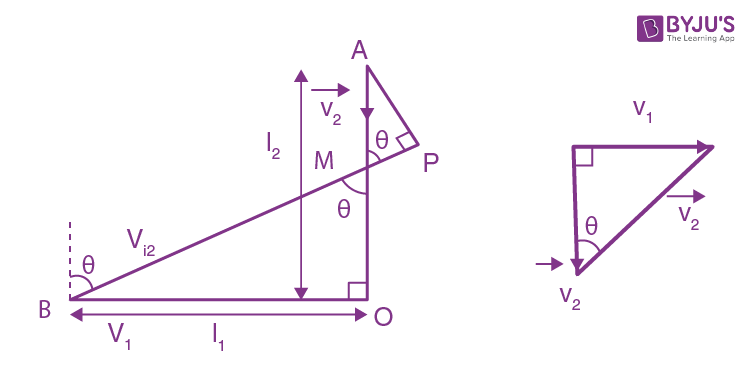From fig(b), v12 = √(v12 + v22) and tan θ = v1/v2

The shortest distance

AP = AM sin θ

= (OA - OM)sin θ

= (l2 - l1 cot θ)sin θ

Or AP = (l2 - l1 v2/v1)v1/ √(v12 + v22)

= v1l2 - v2l1/ √(v12 + v22) (using 1)

The sought time can be obtained directly from the condition that (l1 - v1t)2 + (l2 - v2t)2 is minimum. This gives t = (l1v1 + l2v2)/(v12 + v22)

17. From point A located on a highway (Fig. 1.2) one has to get by car as soon as possible to point B located in the field at a distance l from the highway. It is known that the car moves in the field η times slower than on the highway. At what distance from point D one must turn off the highway?

Solution:

1. Let the car turn off the highway at a distance x from the point D. So, CD = x, and if the speed of the car in the field is v, then the time taken by the car to cover the distance AC = AD - x on the highway

t1 = (AD - x)/ηv ..(1)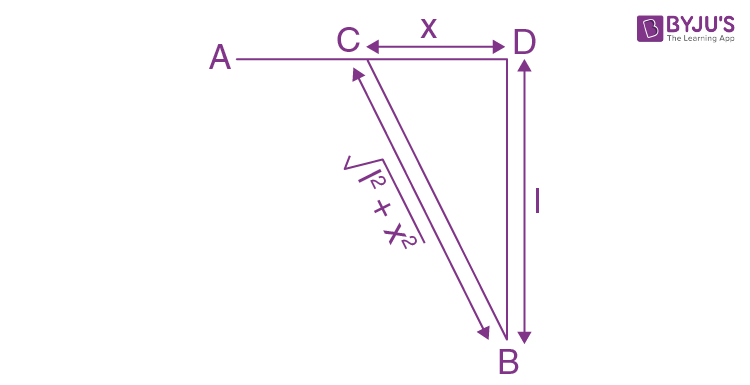The time taken to travel the distance CB in the field

t2 = √(l2 + x2)/v ..(2)

So the total time elapsed to move the car from point A to B

t = t1 + t2

= (AD - x)/ηv + √(l2 + x2)/v

For t to be minimum

dt/dx = 0 or (1/v)(-1/η + x/√(l2 + x2)) = 0

Or η2x2 = l2 + x2 or x = l/√(η2 - 1)

18. A point travels along the x axis with a velocity whose projection vx is presented as a function of time by the plot in Fig. 1.3.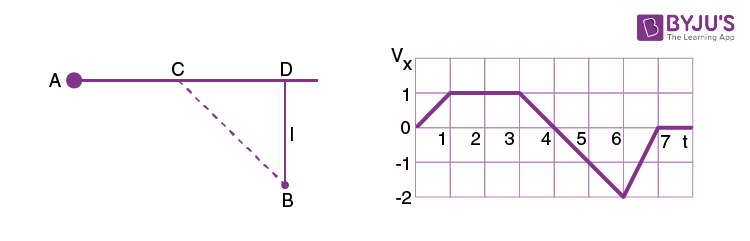Assuming the coordinate of the point x = 0 at the moment t = 0, draw the approximate time dependence plots for the acceleration wx, the x coordinate, and the distance covered s.

Solution:

1. To plot x(t), s(t) and w x (t) let us partition the given plot vx(t) into five segments (for detailed analysis) as shown in the figure.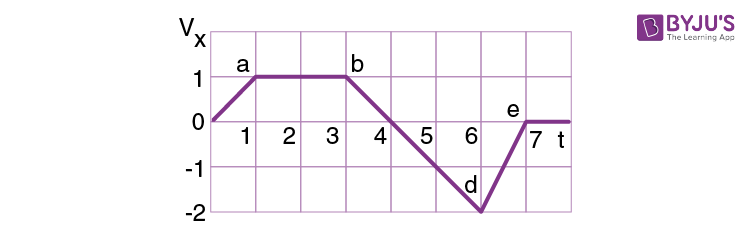For the part oa: wx = 1 and vx = t = v

Thus ∆x1(t) = ∫vx dt = ∫0tdt = t2/2 = s1(t)

Putting t = 1, we get, ∆x1 = s = ½ unit

For the part ab:

wx = 0 and vx = v = constant = 1

Thus ∆x2 (t) = ∫vx dt = ∫1t dt = (t - 1) = s2(t)

Putting t = 3, ∆x2 = s2 = 2 unit

For part b4: wx = 1 and vx = 1 - (t - 3) = 4 - t = v

Thus ∆x3(t) = ∫3t (4 - t)dt = 4t - t2/2 - 15/2 = s3(t)

Putting t = 4, ∆x3 = x3 = 1/2 unit

For part 4d: vx= -1 and vx = -(1-4) = 4 -1

So v = |vx| = t - 4 for t> 4

Thus ∆x4(t) = ∫4t (1 - t)dt = 4t - t2/2 8= s3(t)

Putting t = 6, ∆x4 = -1

Similarly s4(t) = ∫|vx| dt = ∫4t(t - 4)dt = t2/2 - 4t + 8

Putting t = 6, s4 = 2 unit.

For part d7: wx = 2 and vx = -2 + 2(t - 6) = 2(t -7)

v = |vx| = 2(7-t) for t ← 7

Now ∆x(t) = ∫t62(t - 7) dt = t2 -14t + 48

Putting t = 4, ∆x5 = -1

Similarly s5(t) = ∫t62(7 - t)dt = 14t - t2 - 48

Putting t = 7, s5 = 1

On the basis of these obtained expressions wx(t), x(t) and s(t) plots can be easily plotted as shown in the figure of answer sheet.

19. A point traversed half a circle of radius R = 160 cm during time interval T = 10.0 s. Calculate the following quantities averaged over that time:

1. (a) the mean velocity (v);
2. (b) the modulus of the mean velocity vector |(v)|;
3. (c) the modulus of the mean vector of the total acceleration |(w)|
4. if the point moved with constant tangent acceleration.

Solution:

1. (a) Mean velocity <v> = total distance covered/time elapsed

= s/t

= πR/T

= 50 cm/s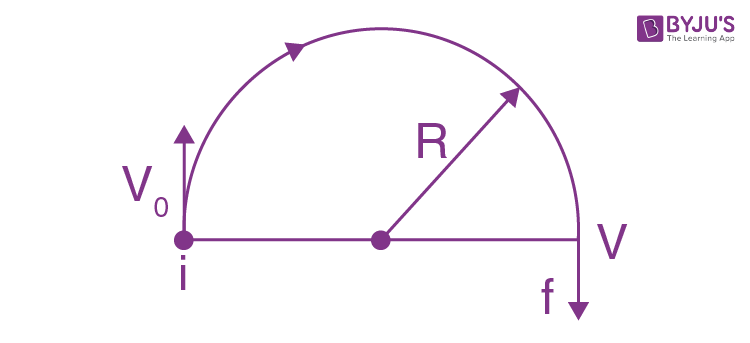(b) Modulus of mean velocity vector = $\left | <\vec{v}> \right |= \frac{\left | \Delta \vec{r} \right |}{\Delta t}$

= 2R/T = 32 cm/s (2)

(c) Let the point moves from i to f along the half circle (fig) and v0 and v be the speed at the points respectively.

We have dv/dt = wt

Or v = v0 + wtt (as wt is constant, according to the problem)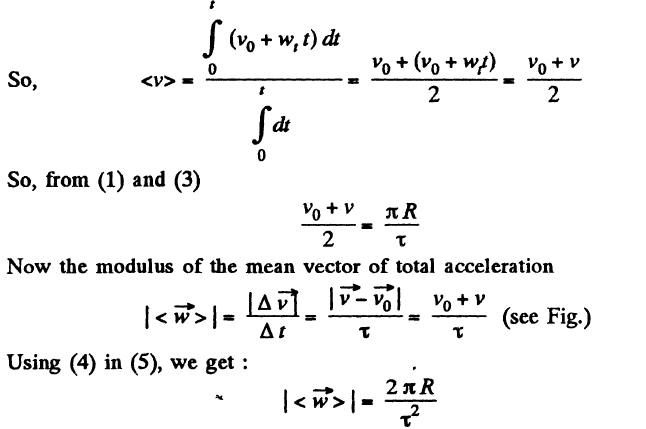20. A radius vector of a particle varies with time t as r = at (1 - αt), where a is a constant vector and α is a positive factor.

Find:

1. (a) the velocity v and the acceleration w of the particle as functions of time;
2. (b) the time interval ∆t taken by the particle to return to the initial points, and the distances covered during that time.

Solution:

1. (a) we have $\vec{r}= \vec{a}t(1-\alpha t)$

So, $\vec{v}= \frac{d\vec{r}}{dt}$

= $= \vec{a}(1-2\alpha t)$

And $\vec{w}=\frac{d\vec{v}}{dt}$

= $= -2\alpha \vec{a}$

(b) from the equation $\vec{r}= \vec{a}t(1-\alpha t)$

$\vec{r}=0$, at t = 0 and also at t = ∆t = 1/α

So the sought time ∆t = 1/α

As $\vec{v}=\vec{a}(1-2\alpha t)$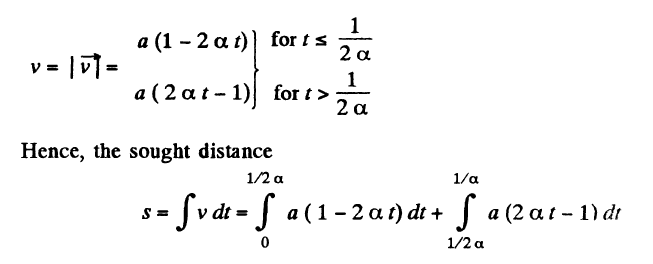Simplifying, we get s = a/2α

21. At the moment t = 0 a particle leaves the origin and moves in the positive direction of the x axis. Its velocity varies with time as v = v0 (1 - t/T), where v0 is the initial velocity vector whose

modulus equals vo = 10.0 cm/s; T = 5.0 s. Find:

1. (a) the x coordinate of the particle at the moments of time 6.0, 10, and 20 s;
2. (b) the moments of time when the particle is at the distance 10.0 cm from the origin;
3. (c) the distance s covered by the particle during the first 4.0 and 8.0 s; draw the approximate plot s(t).

Solution:

1. As the particle leaves the origin at t = 0

So ∆x = x = ∫vx dt

As $\vec{v}=\vec{v}_{0}(1-\frac{t}{T})$

Where vector v0 is directed towards the +ve x axis

So vx = v0(1 - t/T)

From (1) and (2)

x = ∫0tv0(1 - t/T)dt = v0t(1-t/2T)

Hence x coordinate of the particle at t = 6s

x = 10×6(1-6/2×5)

= 24 cm

=0.24 m

Similarly at t = 10 s

x = 10×10(1-10/2×5)

= 0

And at t = 20 s

x = 10 ×20(1-20/2×5)

= -200 cm

= -2 m

b) At the moments the particle is at a distance of 10 cm from the origin, x = ±10 cm

Putting x = +10 in eq (3)

10 = 10t(1 - t/10) or t2 - 10t + 10 = 0

So t = 10±√(100 - 40)/2

= 5±√15 s

Now putting x = -10 in eqn (3)

-10 = 10(1-t/10)

On solving, t = 5±√35 s

As t cannot be negative, so

t = (5+√35) s

As t cannot be negative, so

t = 5 + √35 s

Hence the particle is at a distance of 10 cm from the origin at three moments of time:

t = 5±√15 s, 5+√35 s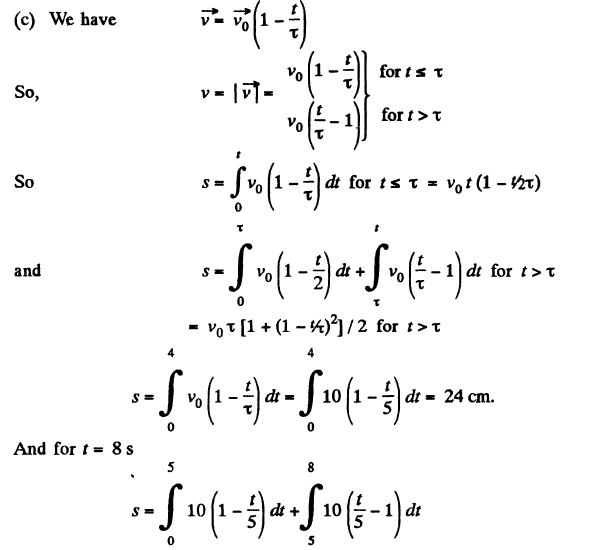On integrating and simplifying, we get s = 34 cm

On the basis of eqn (3) and (4), x(t) and s(t) plots can be drawn as shown in the answer sheet.

22. The velocity of a particle moving in the positive direction of the x axis varies as v = α√x, where α is a positive constant.

Assuming that at the moment t = 0 the particle was located at the point x = 0, find:

1. (a) the time dependence of the velocity and the acceleration of the particle;
2. (b) the mean velocity of the particle averaged over the time that the particle takes to cover the first s metres of the path.

Solution:

1. (a) As particle is in unidirectional motion it is directed along the x axis at t = 0, x = 0 axis all the time.

So ∆x = x = s, and dv/dt = w

therefore , v = α√x = α√s

Or w = dv/dt

= (α/2√s)(ds/dt)

= α/2√s

= αv/2√s

= α2/2

As w = dv/dt

= α2/2

On integrating $\int_{0}^{v}dv=\int_{0}^{t}\frac{\alpha ^{2}}{2}$

Or v = α2t/2

(b) Let s be the time to covers first s m of the path. From the eq.

s = ∫v dt

s = ∫0t α2/2 dt

= (α2/2) (t2/2) using (2)

Or t = 2√s/α

The mean velocity of the particle

v = ∫v(t)dt/∫dt

= α√s/2

23. A point moves rectilinearly with deceleration whose modulus depends on the velocity v of the particle as w = a√v, where a is a positive constant. At the initial moment the velocity of the point is equal to v0. What distance will it traverse before it stops? What time will it take to cover that distance?

Solution:

1. According to the problem

-v dv/ds = a√v (as v decreases with time)

Or $\int_{v_{0}}^{0}\sqrt{v}dv=a\int_{0}^{s}ds$

On integrating we get s = 2v03/2/3a

Again according to the problem -dv/dt = a√v

Or -dv/√v = a dt

Or, $\int_{v_{0}}^{0}\frac{dv}{\sqrt{v}}=a\int_{0}^{t}dt$

Thus t = 2√v0/a

24. A radius vector of a point A relative to the origin varies with time t as r = ati - bt2j, where a and b are positive constants, and i and j are the unit vectors of the x and y axes. Find:

1. (a) the equation of the point's trajectory y (x); plot this function;
2. (b) the time dependence of the velocity v and acceleration w vectors, as well as of the moduli of these quantities;
3. (c) the time dependence of the angle α between the vectors w and v;
4. (d) the mean velocity vector averaged over the first t seconds of motion, and the modulus of this vector.

Solution:

1.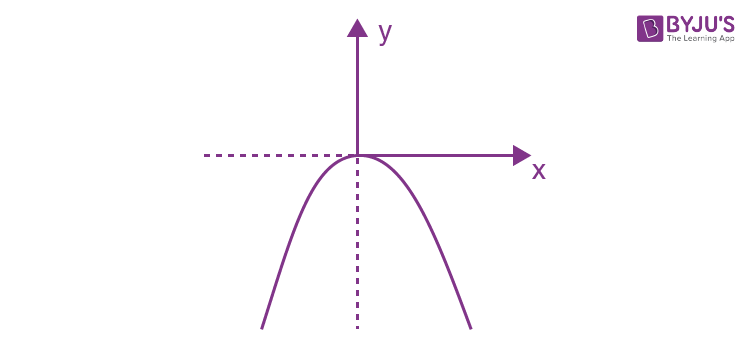(a) As $\vec{r}=at\vec{i}-bt^{2}\vec{j}$

x = at, y = -bt2

And therefore y = -bx2/a2

which is Eq. of a parabola, whose graph is shown in the fig.

(b) As $\vec{r}=at\vec{i}-bt^{2}\vec{j}$

$\vec{v}=\frac{d\vec{r}}{dt}=a\vec{i}-2bt\vec{j}$

So v = √(a2 + (2bt)2) = √(a2 + 4b2t2)

Diff. Eq.(1) w.r.t.time, we get

$\vec{w}= \frac{d\vec{v}}{dt}= -2b\vec{j}$

So |w| = 2b

(c) cos α = $\frac{\vec{v}.\vec{w}}{vw}$

cos α = 2bt/√(a2+4b2t2)

So tan α = a/2bt

Or α = tan-1 (a/2bt)

(d) the mean velocity vector

$\vec{v} = \frac{\int \vec{v}dt}{\int dt}$

= $\frac{\int_{0}^{t}(a\vec{i}-2bt\vec{j})dt}{t}=a\vec{i}-bt\vec{j}$

Hence $\left | \vec{v} \right |= \sqrt{a^{2}+(-bt)^{2}}$

= √(a2 + b2t2)

25. A point moves in the plane xy according to the law x = at, y = at (1-αt), where a and α are positive constants, and t is time. Find:

1. (a) the equation of the point's trajectory y (x); plot this function;
2. (b) the velocity v and the acceleration w of the point as functions of time;
3. (c) the moment t0 at which the velocity vector forms an angle π/4 with the acceleration vector.

Solution:

1. (a) We have x = at and y = αt (1- αt) ..(1)

Hence, y (x) becomes,

y = (ax/a)(1 - αx/a)

= x - αx2/a (parabola)

(b) Differentiating Eq. (1) we get

vx = a and vy = a(1 - 2αt)

So v = √(vx2 + vy2)

= a√(1 + (1 - 2αt)2)

Diff. Eq. (2) w.r.t.time

wx = 0 and wy = -2aα

So w = √(wx2 + wy2)

= 2aα

(c) From Eqs. (2) and (3)

We have $\vec{v}= a\vec{i}+a(1-2\alpha t)\vec{j}$

And $\vec{w}= 2a\alpha \vec{j}$

So cos π/4 = 1/√2 = $\frac{\vec{v}.\vec{w}}{vw}$

= -a(1 - 2αt0)2aα/a√(1 + (1 - 2αt0)22aα

On simplifying

1 - 2αt0 = ±1

As, t ≠ 0, t0 = 1/α

26. A point moves in the plane xy according to the law x = a sin ωt, y = a (1 - cos ωt), where a and ω are positive constants. Find:

1. (a) the distance s traversed by the point during the time T ;
2. (b) the angle between the point's velocity and acceleration vectors.

Solution:

1. Differentiating motion law : x = a sin ωt , y = a (1 - cos ωt ), with respect to time, vx= a ω cos ωt, vy = a ω sin ωt

So $\vec{v}=a\omega \cos \omega t\vec{i}+a\omega \sin \omega t\vec{j}$ ..(1)

And v = aω = constant ..(2)

Differentiating Eq. (1) with respect to time

$\vec{w}=\frac{d\vec{v}}{dt}=-a\omega ^{2}\sin \omega t\vec{i}+a\omega ^{2}\cos \omega t\vec{j}$ ..(3)

(a) the distance s traversed by the point during the time T is given by

s = ∫0Tv dt = ∫0Taω dt = aωT (using 2)

(b) Taking inner product of vector v and vector w

We get $\vec{v}.\vec{w}= (a\omega \cos \omega t\vec{i}).(a\omega ^{2}\sin \omega t(-\vec{i})+a\omega ^{2}\cos \omega t(-\vec{j}))$

So $\vec{v}.\vec{w}$ = -a2ω2 sin ωt cos ωt + a2 ω3 sin ωt cos ωt = 0

Thus $\vec{v}$ perpendicular to $\vec{w}$

So the angle between velocity vector and acceleration vector equals π/2.

27. A particle moves in the plane xy with constant acceleration w directed along the negative y axis. The equation of motion of the particle has the form y = ax - bx2, where a and b are positive constants. Find the velocity of the particle at the origin of coordinates.

Solution:

1. According to the problem

$\vec{w}=w(-\vec{j})$

So, wx = dvx/dt = 0 and wy = dvy/dt = -w ..(1)

Differentiating Eq. of trajectory, y = ax - bx2, with respect to time

dy/dx = a dx/dt - 2bx dx/dt ..(2)

So, dy/dx at (x = 0) = a dx/dtat x = 0

Differentiating w.r.t.time

d2y/dx2 = ad2x/dt2 - 2b(dx/dt)2 - 2bx d2x/dt2

Or -w = a(0) - 2b(dx/dt)2 - 2bx(0) (using 1)

Or dx/dt = √(w/2b) (using (1)) (3)

Using (3) in (2) dy/dtx=0 = a √(w/2b) ..(4)

Hence the velocity of the particle at the origin

v = √((dx/dt)2x=0 + (dy/dt)2x=0 )

= √((w/2b) + (a2w/2b))

Hence v = √((w/2b)(1+a2))

28. A small body is thrown at an angle to the horizontal with the initial velocity vo. Neglecting the air drag, find:

1. (a) the displacement of the body as a function of time r (t);
2. (b) the mean velocity vector (v) averaged over the first t seconds and over the total time of motion.

Solution:

1.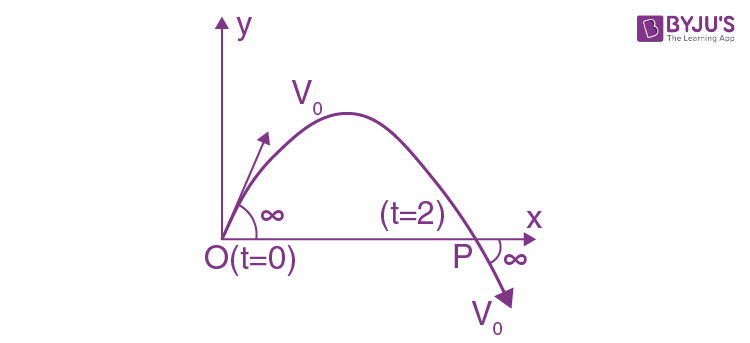As the body is under gravity of constant acceleration g, its velocity vector and displacement vectors are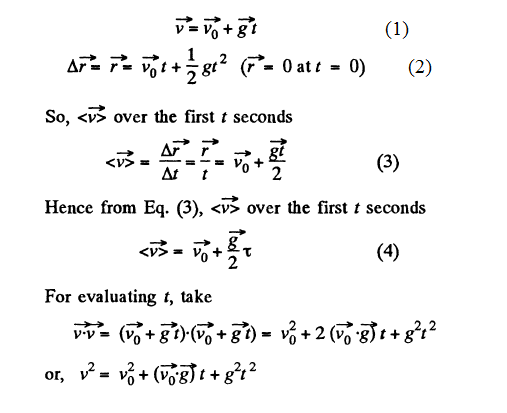But we have v = v0 at t = 0 and also at t = T (also from energy conservation)

Hence using this property in Eq. 5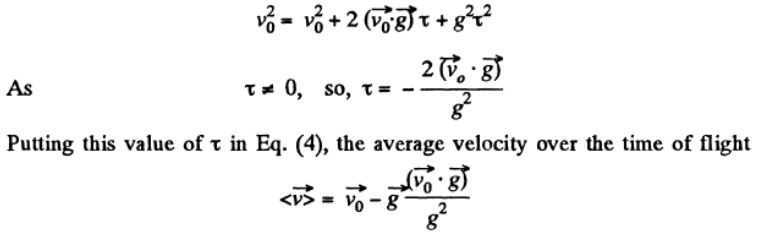29. A body is thrown from the surface of the Earth at an angle α to the horizontal with the initial velocity v0. Assuming the air drag to be negligible, find:

1. (a) the time of motion;
2. (b) the maximum height of ascent and the horizontal range; at what value of the angle a they will be equal to each other;
3. (c) the equation of trajectory y (x), where y and x are displacements of the body along the vertical and the horizontal respectively;
4. (d) the curvature radii of trajectory at its initial point and at its peak.

Solution:

1.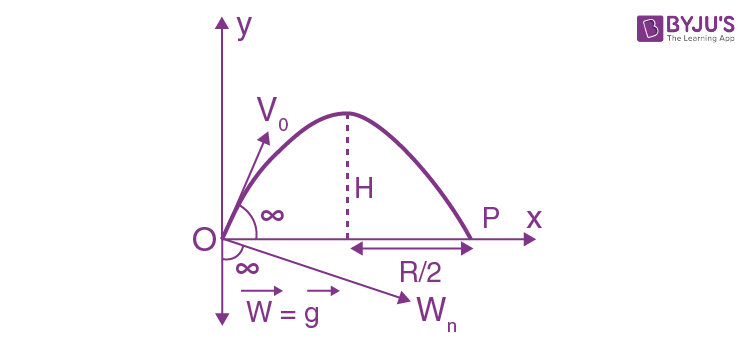The body thrown in air with velocity v0 at an angle α from the horizontal lands at point P on the Earth's surface at same horizontal level (Fig.). The point of projection is taken as origin, so, Δx = x and Δy = y

(a) from the eq. ∆y = v0yt + ½ wyt2

0 = v0 sin αT - ½ gT2

At T not equal 0, so time of motion T = 2v0 sin α/g

(b) At the max. Height of ascent, vy = 0

So from the Eq. vy2 = v0y2 + 2wy∆y

0 = (v0sin α)2 - 2gH

Hence maximum height H = v02 sin2 α/2g

During the time of motion the net horizontal displacement or horizontal range, will be obtained by the equation

∆x = v0x t + ½ wxT2

Or R = v0 cos αT - ½ (0)T2

= v0cos αT

= v02sin 2α/g

When R = H

v02sin2α/g = v02sin2α/2g

Or tan α = 4

So α = tan-1 4

(c) For the body, x(t) and y(t) are x = v0cos αt

And y = v0 sin αt - ½ gt2

Hence putting the value of t from (1) and (2) we get,

y = v0 sin α(x/v0 cos α) - ½ g(x/v0cos α)2 = x tan α - gx2/2v02cos2α

Which is the sought equation of trajectory i.e y(x)

(d) As the body thrown in air follows a curve, it has some normal acceleration at all the moments of time during it’s motion in air. At the initial point (x = 0, y = 0), from the equation

wn = v2/R (where R is the radius of curvature)

g cos α = v02/R0 (see fig)

Or R0 = v02/g cos α

At the peak point vy = 0, v = vx = v0 cos α and the tangential acceleration is zero.

Now from the Eq. wn = v2/R

g = v02 cos2α/R, or R = v02 cos2α/g

Note: We may use the formula of curvature radius of a trajectory y(x)n to solve part (d)

R = [1 + (dy/dx)2]3/2/|d2y/dx2|